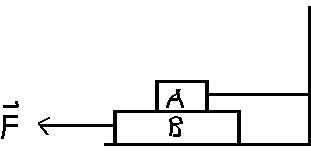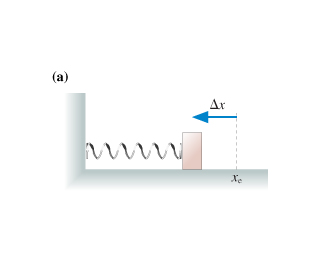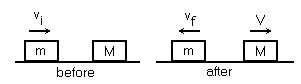Ask question

# Block A in the fig. 5.58 weighs 1.20N and Block B weighs3.60N.# Block A in the fig. 5.58 weighs 1.20N and Block B weighs3.60N.

Question
Otherasked 2020-10-18

Block A in the fig. 5.58 weighs 1.20N and Block B weighs3.60N. The Coefficient of kinetic friction between all surfaces is0. 300. Find the magnitude of the horizontal force F necessary to drag black B to the left at a constant speed a) if A rest on B and moves with it (fig. 5.28am). b) If A is held at rest.## Answers (1)2020-10-19
a) The most direct way to do part (a) is toconsider the blocks as a unit, with total weight 4.80 N. Then thenormal force between block B and the lower surface is 4.80N, and the friction force that must be overcome by the force F is $$\displaystyle{.3}\cdot{4.8}={1.44}{N}\to{t}{h}{r}{e}{e}{f}{i}{g}{u}{r}{e}{s}.{b}{)}{T}{h}{e}{\left\|{a}\right\|}{l}{f}{\quad\text{or}\quad}{c}{e}{b}{e}{t}{w}{e}{e}{n}{b}{l}{o}{c}{k}{B}{\quad\text{and}\quad}{t}{h}{e}{l}{o}{w}{e}{r}{s}{u}{r}{f}{a}{c}{e}{i}{s}{s}{t}{i}{l}{l}{4.80}{N},{b}{u}{t}{\sin{{c}}}{e}{b}{l}{o}{c}{k}{A}{i}{s}{m}{o}{v}\in{g}{r}{e}{l}{a}{t}{i}{v}{e}\to{b}{l}{o}{c}{k}{B},{t}{h}{e}{r}{e}{i}{s}{a}{\mathfrak{{i}}}{c}{t}{i}{o}{n}{f}{\quad\text{or}\quad}{c}{e}{b}{e}{t}{w}{e}{e}{n}{t}{h}{e}{b}{l}{o}{c}{k}{s},{o}{f}{m}{a}{g}{n}{i}{t}{u}{d}{e}{P}{S}{K}{.3}\cdot{1.2}={.360}$$ N so the total frictionforce F must overcome 1.44+.36=1.8N

### Relevant Questionsasked 2021-02-16
A 5 kg block of aluminum is heated from 20 degrees Celsius to90 degrees Celsius at atmospheric pressure. Find a)the workdone by the aluminum b) the amount of energy transferred to it byheat, and c) the increase in its internal energy?asked 2021-02-21
A 15.0 kg block is dragged over a rough, horizontal surface by a70.0 N force acting at 20.0 degree angle above the horizontal. The block is displaced 5.0 m, and the coefficient of kinetic friction is 0.3. Find the work done on the block by ; a) the 70.0 N force,b) the normal force, and c) the gravitational force. d) what is the increase in the internal energy of the block-surface system due to friction? e) find the total change in the kinetic energy of the block.asked 2021-03-24
A 2.4-kg object is attached to a horizontal spring of forceconstant k=4.5 kN/m. The spring is stretched 10 cm fromequilibrium and released. Find (a) the frequency of themotion, (b) the period, (c) the amplitude, (d) the maximum speed,and (e) the maximum acceleration. (f) When does the objectfirst reach its equilibrium position? What is itsacceleration at this time?
Two identical blocks placed one on top of the other rest on africtionless horizontal air track. The lower block isattached to a spring of spring constant k= 600 N/m. Whendisplaced slightly from its equilibrium position, the systemoscillates with a frequency of 1.8 Hz. When the amplitude ofoscillation exceeds 5 cm, the upper block starts to slide relativeto the lower one. (a) What are the masses of the twoblocks? (b) What is the coefficient of static frictionbetween the two blocks?asked 2021-02-19
A 10 kg objectexperiences a horizontal force which causes it to accelerate at 5 $$\displaystyle\frac{{m}}{{s}^{{2}}}$$, moving it a distance of 20 m, horizontally.How much work is done by the force?
A ball is connected to a rope and swung around in uniform circular motion.The tension in the rope is measured at 10 N and the radius of thecircle is 1 m. How much work is done in one revolution around the circle?
A 10 kg weight issuspended in the air by a strong cable. How much work is done, perunit time, in suspending the weight?
A 5 kg block is moved up a 30 degree incline by a force of 50 N, parallel to the incline. The coefficient of kinetic friction between the block and the incline is .25. How much work is done by the 50 N force in moving the block a distance of 10 meters? What is the total workdone on the block over the same distance?
What is the kinetic energy of a 2 kg ball that travels a distance of 50 metersin 5 seconds?
A ball is thrown vertically with a velocity of 25 m/s. How high does it go? What is its velocity when it reaches a height of 25 m?
A ball with enough speed can complete a vertical loop. With what speed must the ballenter the loop to complete a 2 m loop? (Keep in mind that the velocity of the ball is not constant throughout the loop).asked 2021-02-23The spring in the figure (a) is compressed by length delta x . It launches the block across a frictionless surface with speed v0. The two springs in the figure (b) are identical to the spring of the figure (a). They are compressed by the same length delta x and used to launch the same block. What is the block's speed now?asked 2021-02-14
Electrocardiographs are often connected as shown in Fig.19-55. The leads are said to be capacitively coupled. A timeconstant of 3.0s is tyical and allows rapid changes in potential tobe recorded accurately. If C = 3.0?F, What value must R have?asked 2021-03-26
A box weighing 70 N rests on a table. A rope tied to the box runs vertically upward over a pulley and a weight is hung from the other end. Determine the force that the table exerts on the box if the weight hanging on the other side of the pulley weighs a) 30 N b) 60 N and c) 90 Nasked 2021-05-01
A uniform door (0.81m wide and 2.1m hight) weighs 140N and ishung on two hinges that fasten the long left side of the door to averticle wall. The hinges are 2.1m apart. Assume that thelower hinge bears all the weight of the door. Find themagnitude and direction of the horizontal component of the forceapplied to the door by (a) the upper hinge and (b) the lowerhinge. Determine the magnitude and direction of the forceapplied by the door to (c) the upper hinge and (d)the lower hinge.asked 2021-03-17
The person weighs 170 lb. Each crutch makes an angle of 22.0 with the vertical. Half of the person's weight is supported by the cruches, the other half by the vertical forces exerted by the roundon his feet. Assuming that he is at rest and that the force exerted by the ground on the crutches acts along the crutches,determine
a) the smallest possible coefficient of friction between crutches and ground and
b) the magnitude of the compression force supported by each crutch.asked 2021-02-23A block of mass m=3.6 kg, moving on africtionless surface with a speed $$\displaystyle{v}_{{1}}={9.3}$$ m/s makes a perfectly elastic collision with a block of mass Mat rest. After the collision, the 3.6 kg block recoils with a speed of $$\displaystyle{v}_{{1}}={2.7}$$ m/s in figure, the speed of the vlock of mass M after the collision is closest to:
a. 9.3 m/s
b. 6.6 m/s
c. 8.0 m/s
d. 10.7 m/s
e. 12.0 m/s
...